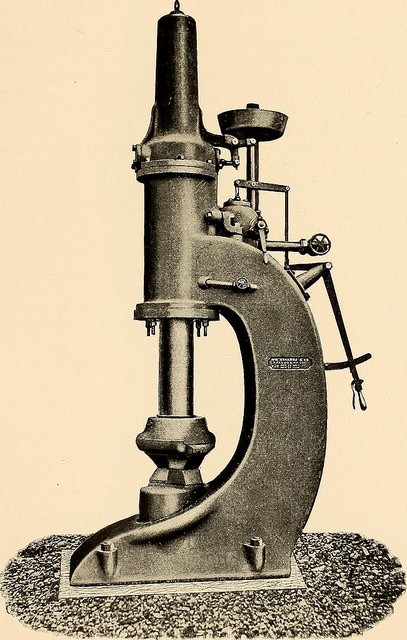# MTBF is a Statistic, Not the Only OneWe often face just a sample of life data with the request of estimating the reliability of the system. Or, we have a touch of test results and want to know if the product is reliability enough, yet. Or, we gather repair times to grapple with spares stocking.

We need to know the reliability. We need to know the number.

MTBF (or close cousin MTTF) is just that number. It is easy to calculate. A higher number means the system is more reliable. And, the metrics are in the units of time, often hours, which is easy to understand (and misunderstand).

In early chapters of reliability engineering books, or in introduction to reliability, we learn about the exponential distribution and the population parameter, theta. We also learn about the sample statistics which provides an unbiased estimated for the population parameter. In both cases, MTBF, or the mean time between failure, is the one value we have to master.

## Other Statistics

Reliability is pretty easy using just one statistic. One calculation, one number, and we’re done.

Then a couple of things start to happen.

First, we notice that the actual time to failure behavior is not predicted, nor follows, the expected pattern when using just MTBF and the exponential distribution. The average time to fail changes as the system ages. We find that we run out of spares based on calculations using MTBF as the parts fail more and more often.

Second, we learn just a little more. We turn the page in the book or attend another webinar. We hear about another distribution commonly used in reliability engineering. The Weibull distribution. But, wait, hold on there. The Weibull has two and sometimes three parameters. I’ll need to learn about plotting, censored data, regression analysis, goodness of fit, confidence intervals, and a bunch of statistical methods.

Life was good with just one statistic.

Actually, when using even just the one statistic, MTBF, we also should have been

• Checking assumptions
• Fitting the data to the exponential distribution function
• Evaluating the goodness of fit
• Calculating confidence bounds
• And, using those other statistical methods

In order to understand and use our sparse and expensive datasets, we need to use the tools found in the statistics textbooks.

Yes, the Weibull distribution has two or three parameters, thus we need to evaluate how well our statistics describe the data in a more rigorous way. And, we learn so much more. For example, we can model and predict a system with decreasing or increasing failure rates over time. We can estimate the number of required spares next year with a bit more accuracy then using just MTBF.

There are more benefits. Have you advanced past the basic introduction and embraced the use of reliability statistics? How’s it going and what challenges are you facing?